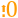# Round Up

 Block Group: Number Formatting Icon:The Round Up block rounds a number up, away from zero if positive and toward zero if negative.

For information on using dataflow blocks, see Dataflow.

For accepted and excluded arguments of Number Formatting blocks, see Number Formatting.

## Input/Output Properties

The following properties of the Round Up block can take input and give output.

• input (number)
• precision (number)

input specifies any real number that you want rounded up.

precision specifies the number of digits after the decimal to round the number to. A value of 0, less than 0, or null rounds the number up to an integer.

## Output Property

The following property of the Round Up block can give output but cannot take input.

• output (number)

output returns the rounded-up number.

## Example

The following image shows an example of the Round Up block. In this example, the number 3.2 is rounded up to an integer.